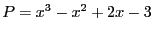Next: Bounds on the product Up: Kantorovitch theorem Previous: Implementation   Contents

Example

Let, Input be 1.2 and the procedure:

Coeff(1)= -3;Coeff(2)=2;Coeff(3)=-1;Coeff(4)=1;
Num=Kantorovitch(3,Coeff,1.2,&eps);
Coeff_App(1)= INTERVAL(-3.1,-2.9);Coeff_App(2)=INTERVAL(1.9,2.1);
Coeff_App(3)=INTERVAL(-1.1,-0.9);Coeff_App(4)=INTERVAL(0.9,1.1);
Num=Kantorovitch(3,Coeff_App,1.2,&eps);
In the first case Kantorovitch returns 1 and has determined that there is an unique solution in the interval [1.04082,1.35918]. Using Newton method we find the root 1.27568220371567 with residue 2.80147904874184e-10. For the interval polynomial Kantorovitch returns also 1 and find an unique solution in [1.05718,1.34282].

Jean-Pierre Merlet 2012-12-20